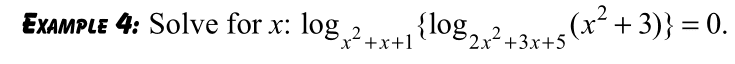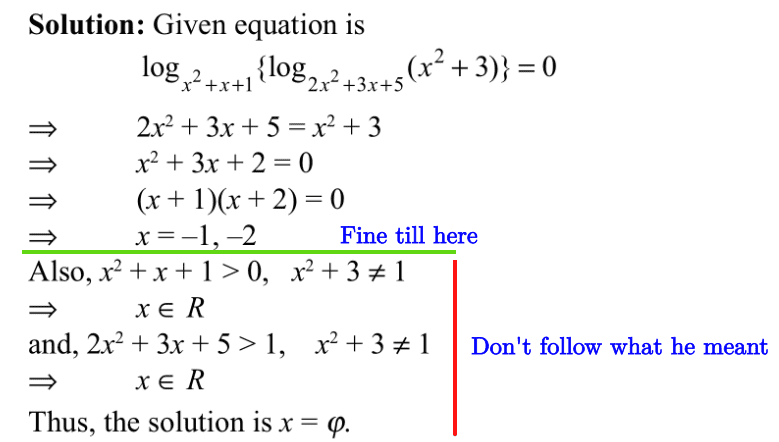# Solving a nested logarithmic equation

brotherbobby
Homework Statement:
Solve for ##x## : ##\boldsymbol{\log_{x^2+x+1}\{\log_{2x^2+3x+5}(x^2+3)\}=0}##
Relevant Equations:
1. Given ##\log_b a= x##, we have the requirements that (1) ##a>0##, (2) ##b>0\; \text{and}\; b\ne 1## and (3) that all ##a,b,x \in \mathbb{R}##
2. If ##\log_{f(x)} g(x) = 0\Rightarrow g(x) = 1\;\forall x##
3. If ##\log_{f(x)} g(x) = 1\Rightarrow g(x) = f(x)\;\forall x##Problem statement :
Let me copy and paste the problem on the right as it appears in the text.

Solution : Using the Relevant Equations (2) and (3) above, we can claim that

\begin{align*}
&\log_{2x^2+3x+5}(x^2+3)=1\\
&\Rightarrow x^2+3 = 2x^2+3x+5\\
&\Rightarrow x^2+3x+2=0\\
&\Rightarrow (x+1)(x+2)=0\\
&\end{align*}

We have three functions to consider for conditions satisfying positivity, as outlined in Relevant Equations (1) above, for the two (underlined) solutions just obtained.

1. ##x^2+3## : Both solutions satisfy this function being greater than zero.
2. ##2x^2+3x+5## : Likewise, both solutions satisfy the requirement of this "base" function for being greater than zero and not equal to one.
3. ##x^2+x+1## : For ##x=-1##, this function is one, which is invalid. Hence this solution has to be discarded. However, for ##x=-2## this function is greater than zero and not one; so this solution holds good.

Answer : ##\Large{\boxed{x = -2}}. ##

Issue : The author says no answer for ##x## satisfies the logarithmic equation, giving ##x=\varnothing##. I copy and paste his solution below :Doubt : Is the author mistaken? Am I? A hint would be most welcome.

Last edited:

Mentor
Your answer looks fine to me. If x = -1, the outer log is base-1, which we can't have.
If x = -2, we have this:
##\log_{x^2 + x + 1}[\log_{2x^2 + 3x + 5}(x^2 + 3)] = \log_3[\log_7(7)] = \log_3(1) = 0##

I don't following the author's work at all, particularly where he writes ##x \in R## a couple of times, and then concludes that the solution set is empty.

•scottdave
brotherbobby
Your answer looks fine to me. If x = -1, the outer log is base-1, which we can't have.
If x = -2, we have this:
##\log_{x^2 + x + 1}[\log_{2x^2 + 3x + 5}(x^2 + 3)] = \log_3[\log_7(7)] = \log_3(1) = 0##

I don't following the author's work at all, particularly where he writes ##x \in R## a couple of times, and then concludes that the solution set is empty.
Thank you. Yes I checked my answer too. Matches the conditions. Sorry about the text.